## Introduction to R and R studio

### The interface of R studio

The interface of R studio is divided into four parts. The top left block shows the code files. The bottom left block is console, where the code is excuted and the result is shown. You can see the files, the plots generated by code, the packages you’ve installed, and the help document in the bottom right block.

### Opening/creating a file

If you would like to open an existing file, click the icon which looks like a folder.

If you would like to create a new file, click the icon at the left of the tool bar. You can choose the type of the file you would like to create. Next we will briefly introduce R script and R markdown.

### R script

R script can only include code and comments. Comments starts following the # sign.

Clicking the run icon or press ctrl and enter at the same time can run the full code file. If you only want to run certain lines, select the lines and click run icon/press ctrl and enter simultaneously.

### R markdown

R markdown is often used when people would like to write a report containing the results generated by R code. To generate a pdf document or html document, click the Knit icon.

The code blocks should always start and end as the following format:

1+1
##  2

In the default setting, both the code block and its results will be shown in the final document. Code blocks have grey background. The results generated will follow right after the corresponding code block.

In the brace, you can set two parameters “echo” and “eval” to change what are included in the final document. If “echo” is set as FALSE, the code block will not appear while it will be excuted and the results will be shown. If “eval” is set as FALSE, the code block will be shown while it will not be excuted.

## Packages

### Installation

install.packages("glasso")

R is an open source software, so there are many useful packages. If it is your fisrt time to use this package, you need to use the code above to install the package.

### Import

library(glasso)

Once the package is successfully installed, it will be there unless the package is uninstalled. However, the package needs to be imported each time when it is used.

## Help document

### Function

help(qnorm)
?(qnorm)

The two lines above are equivalent for searching the usage of a certain function. In the help document, “usage” module shows how the function looks like. The arguments give out the definition of the input parameter of the function. “Value” tells what the function will return.

### Package

help(base)

You can use the same code to reach out to the help document of a package.

## Data import and summary

### Data import

In this section, we will introduce how to import the data in txt or excel document. The data set is the Female Horseshoe Crabs data from Example 1.5 in the book$$^{}$$ , which can be download from the website www.stat.ufl.edu/~aa/glm/data.

#### Txt file

crab_txt = read.table("D:/Stat@UChicago/2021Spring/consulting/Crabs.txt")
head(crab_txt) #only shows the first 6 rows of the data
##     V1 V2     V3    V4    V5    V6
## 1 crab  y weight width color spine
## 2    1  8  3.050  28.3     2     3
## 3    2  0  1.550  22.5     3     3
## 4    3  9  2.300  26.0     1     1
## 5    4  0  2.100  24.8     3     3
## 6    5  4  2.600  26.0     3     3

To import the data in txt file, we use the function “read.table” where the path of the file is put between the quotation marks. Please note that forward slash should be used in the path rather than the backslash. The data set is named as “crab_txt”, which means crab_txt will refer to this imported data set unless crab_txt is redefined.

You should note that the first row refers to the variables. However, the varaibles are supposed to be the names of columns instead of appearing as the first row. Thus, you can add a parameter “head” after the path of the file to make the modification.

crab_txt = read.table("D:/Stat@UChicago/2021Spring/consulting/Crabs.txt", head = TRUE)
head(crab_txt) #only shows the first 6 rows of the data
##   crab y weight width color spine
## 1    1 8   3.05  28.3     2     3
## 2    2 0   1.55  22.5     3     3
## 3    3 9   2.30  26.0     1     1
## 4    4 0   2.10  24.8     3     3
## 5    5 4   2.60  26.0     3     3
## 6    6 0   2.10  23.8     2     3

Now each column is named by the corresponding variable. The first row becomes the data of the first female crab.

#### Excel file

crab_excel = read.csv("D:/Stat@UChicago/2021Spring/consulting/Crabs.csv", head = TRUE)
head(crab_excel)
##   crab y weight width color spine
## 1    1 8   3.05    NA     2     3
## 2    2 0   1.55  22.5     3     3
## 3    3 9   2.30  26.0     1     1
## 4    4 0   2.10  24.8     3     3
## 5    5 4   2.60  26.0     3     3
## 6    6 0   2.10  23.8     2     3

It is similar to import a excel file using the function “read.csv”.

### The dimension of data

dim(crab_excel)
##  173   6

The function “dim” returns the dimension of the data set. In this example, the return value means this data set has 173 rows and 6 columns.

### Summary of data

The value in the first row and fourth column in the excel file is deleted to better explain how to see missing values.

“summary” is commonly used to take a first look at the data.

summary(crab_excel)
##       crab           y              weight          width           color
##  Min.   :  1   Min.   : 0.000   Min.   :1.200   Min.   :20.00   Min.   :1.000
##  1st Qu.: 44   1st Qu.: 0.000   1st Qu.:2.000   1st Qu.:24.70   1st Qu.:2.000
##  Median : 87   Median : 2.000   Median :2.300   Median :26.05   Median :2.000
##  Mean   : 87   Mean   : 2.919   Mean   :2.423   Mean   :26.23   Mean   :2.434
##  3rd Qu.:130   3rd Qu.: 5.000   3rd Qu.:2.800   3rd Qu.:27.62   3rd Qu.:3.000
##  Max.   :173   Max.   :15.000   Max.   :5.200   Max.   :33.50   Max.   :4.000
##                                                 NA's   :1
##      spine
##  Min.   :1.00
##  1st Qu.:2.00
##  Median :3.00
##  Mean   :2.48
##  3rd Qu.:3.00
##  Max.   :3.00
## 

NA’s shows the number of the missing values in the corresponding column. For example, the first value in the fourth column is missing, so the NA’s for “width” column is 1.

### Missing values

Furthermore, you might care about the location of the missing value in the “width” column. The following line can be used to identify which row the missing value locates.

which(is.na(crab_excel$width)) ##  1 ## Variable definition There are basically two different variable assignment methods: a = 2 b <- 3 They are the same in the above setting. However, median(x = 1:10) ##  5.5 x ## Error in eval(expr, envir, enclos): object 'x' not found In the above case, x is defined with =, and the scope of the variable is local. Let’s see what happens when we switch to <-. median(x <- 1:10) ##  5.5 x ##  1 2 3 4 5 6 7 8 9 10 When using <-, the variable x is globally defined. In most cases, these two assignment methods are the identical, but the R community seems to prefer <- to =. ## Basic Operations a <- 10; b <- 2; a + b ##  12 a - b ##  8 a * b  ##  20 a / b ##  5 ## Data Types ### Vector The following are three different ways to define a vector. vec1 <- c(2,3,2,4,1); vec1 #add the elements in order ##  2 3 2 4 1 vec2 <- 2:6; vec2 #generate a sequence from 2 to 6 by step length 1 ##  2 3 4 5 6 vec3 <- seq(0,10, by=2); vec3 ##generate a sequence from 0 to 10 by step length 2 ##  0 2 4 6 8 10 #### Some useful functions rev(vec1) #reverse a vector ##  1 4 2 3 2 sort(vec1) #sort the elements of a vector ##  1 2 2 3 4 unique(vec1) #delete the repeated elements in a vector ##  2 3 4 1 ### Matrix We introduce how to generate a matrix. x = 1:16 #the elements in the matrix #How to arrange the elements? m1 = matrix(x, nrow = 4); m1 #the matrix has 4 rows, and the elements are arranged by column ## [,1] [,2] [,3] [,4] ## [1,] 1 5 9 13 ## [2,] 2 6 10 14 ## [3,] 3 7 11 15 ## [4,] 4 8 12 16 m2 = matrix(x, nrow = 2, ncol = 8); m2 #the matrix has 2 rows and 8 columns, and the elements are arranged by column ## [,1] [,2] [,3] [,4] [,5] [,6] [,7] [,8] ## [1,] 1 3 5 7 9 11 13 15 ## [2,] 2 4 6 8 10 12 14 16 #### Extract elements from the matrix m1[1,] #the first row ##  1 5 9 13 m1[,3] #the third column ##  9 10 11 12 m1[2,2] #the entry at the second row and second column ##  6 #### Basic matrix operations t(m1) # transpose ## [,1] [,2] [,3] [,4] ## [1,] 1 2 3 4 ## [2,] 5 6 7 8 ## [3,] 9 10 11 12 ## [4,] 13 14 15 16 det(m1) # determinant ##  0 diag(m1) # diagonal elements ##  1 6 11 16 diag(4) # identity matrix of 4 x 4 ## [,1] [,2] [,3] [,4] ## [1,] 1 0 0 0 ## [2,] 0 1 0 0 ## [3,] 0 0 1 0 ## [4,] 0 0 0 1 ### String s = "hello world!"; s ##  "hello world!" # string concatenation s = paste(s, "I'm new to R"); s ##  "hello world! I'm new to R" # string formatting hour = 9; minute = 30; s = sprintf("Hello! It's %d:%d a.m. now", hour, minute); s ##  "Hello! It's 9:30 a.m. now" ### Data Frame # generate a data frame survey <- data.frame("index" = c(1, 2, 3, 4, 5), "age" = c(24, 25, 42, 56, 22)) survey ## index age ## 1 1 24 ## 2 2 25 ## 3 3 42 ## 4 4 56 ## 5 5 22 # extract a column survey$index
##  1 2 3 4 5
survey$age  ##  24 25 42 56 22 ## adding another column survey$edu <- as.factor(c("college", "hs", "hs", "doctorate", "college"))
survey 
##   index age       edu
## 1     1  24   college
## 2     2  25        hs
## 3     3  42        hs
## 4     4  56 doctorate
## 5     5  22   college
## summary of data frame
summary(survey)
##      index        age              edu
##  Min.   :1   Min.   :22.0   college  :2
##  1st Qu.:2   1st Qu.:24.0   doctorate:1
##  Median :3   Median :25.0   hs       :2
##  Mean   :3   Mean   :33.8
##  3rd Qu.:4   3rd Qu.:42.0
##  Max.   :5   Max.   :56.0

## Plots

In this section, we will show how to generate some plots using R code. The built-in data set iris will be used as an example.

Let us first take a look at the iris data set.

data <- iris
head(data)
##   Sepal.Length Sepal.Width Petal.Length Petal.Width Species
## 1          5.1         3.5          1.4         0.2  setosa
## 2          4.9         3.0          1.4         0.2  setosa
## 3          4.7         3.2          1.3         0.2  setosa
## 4          4.6         3.1          1.5         0.2  setosa
## 5          5.0         3.6          1.4         0.2  setosa
## 6          5.4         3.9          1.7         0.4  setosa
summary(data)
##   Sepal.Length    Sepal.Width     Petal.Length    Petal.Width
##  Min.   :4.300   Min.   :2.000   Min.   :1.000   Min.   :0.100
##  1st Qu.:5.100   1st Qu.:2.800   1st Qu.:1.600   1st Qu.:0.300
##  Median :5.800   Median :3.000   Median :4.350   Median :1.300
##  Mean   :5.843   Mean   :3.057   Mean   :3.758   Mean   :1.199
##  3rd Qu.:6.400   3rd Qu.:3.300   3rd Qu.:5.100   3rd Qu.:1.800
##  Max.   :7.900   Max.   :4.400   Max.   :6.900   Max.   :2.500
##        Species
##  setosa    :50
##  versicolor:50
##  virginica :50
##
##
## 

### Types of Plots

• Histograms
• Barplots
• Boxplots
• Scatterplots

#### Histograms

The most basic usage of “hist” function is inputting the data for the histogram. In this setting, the number of the bins will be automatically decided by the function.

hist(data$Sepal.Length)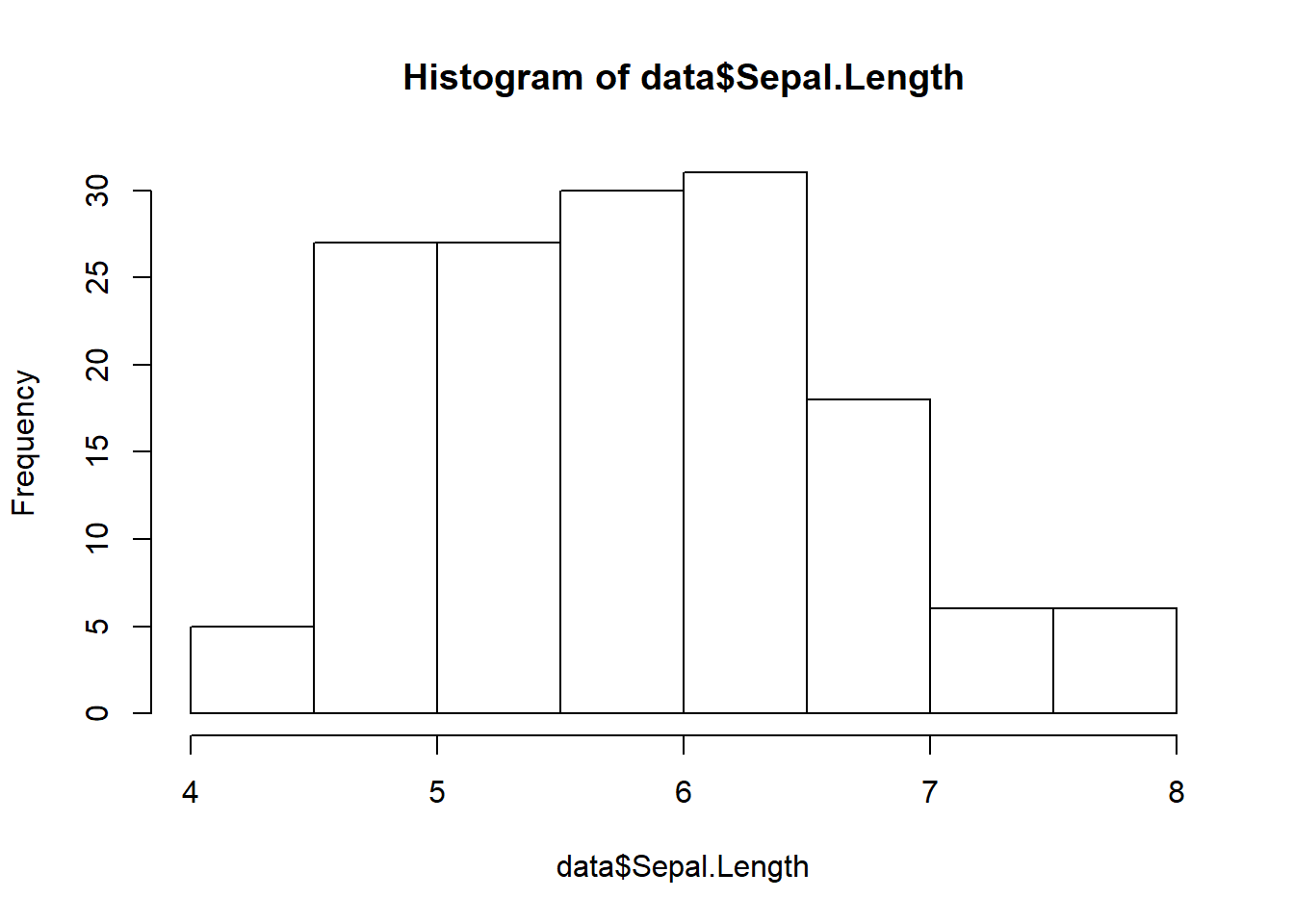There are many parameters to adjust the histogram. Please see the following code block for details. hist(data$Sepal.Length, #the data for histogram
main = "Histogram of Sepal Length", #title of the histogram
xlab = "Sepal Length", #the name of x axis
breaks = 20, #the number of the bins in histogram
col = "grey90", #color of the fillings in the bins
border = "darkblue")#color of the border of the bins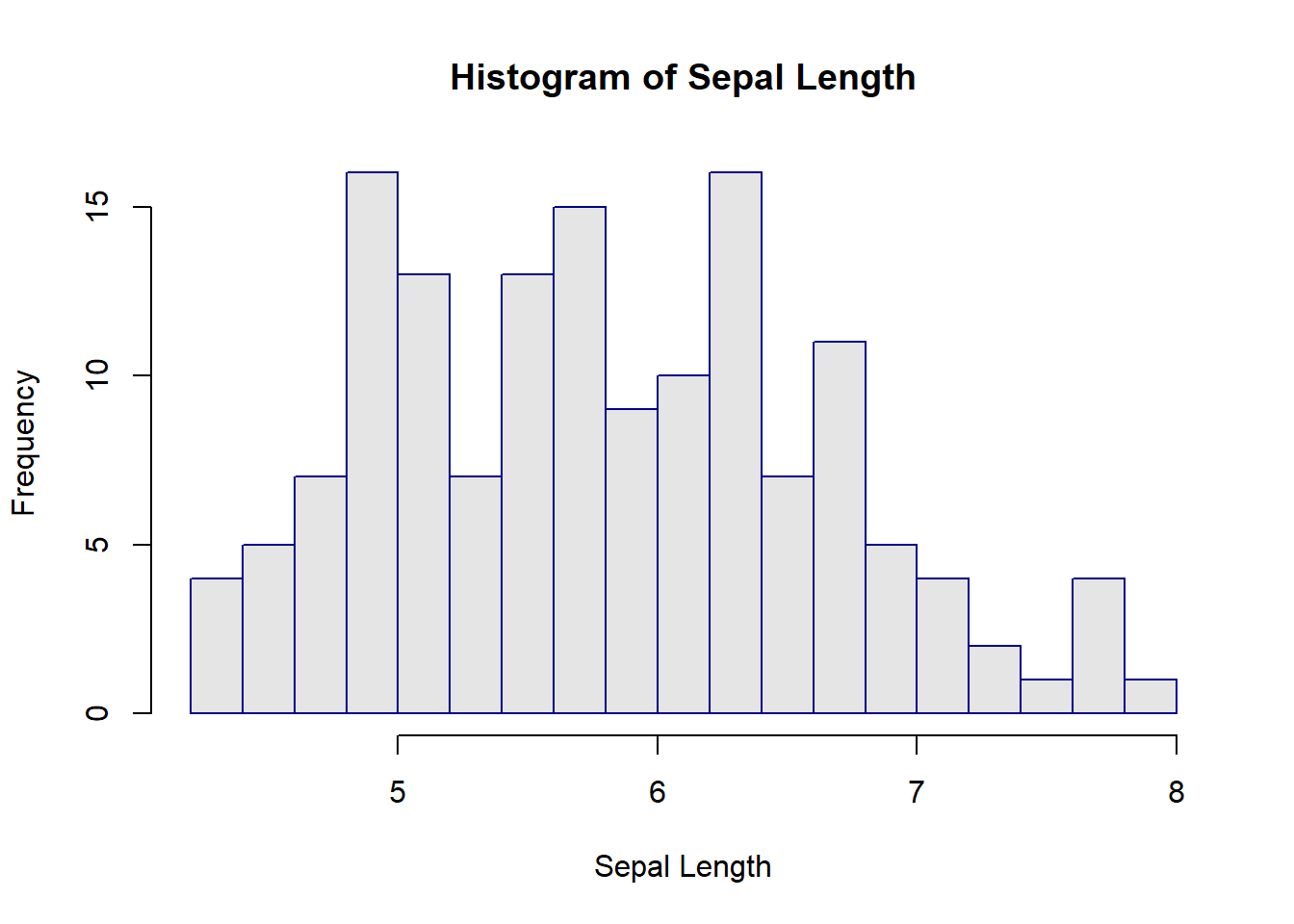* breaks is specific to hist() * By default R will attempt to intelligently guess a good number of breaks * Entering an integer will give a suggestion to R for how many bins to use for the histogram

#### Barplots

Barplots are useful to visualize the summary of a categorical variable

table(data$Species) ## ## setosa versicolor virginica ## 50 50 50 “table” is a function which returns the counts. In the code block above, it shows the numbers of flowers for three species. The most basic usage of “barplot” is entering the counts of all the classes. barplot(table(data$Species))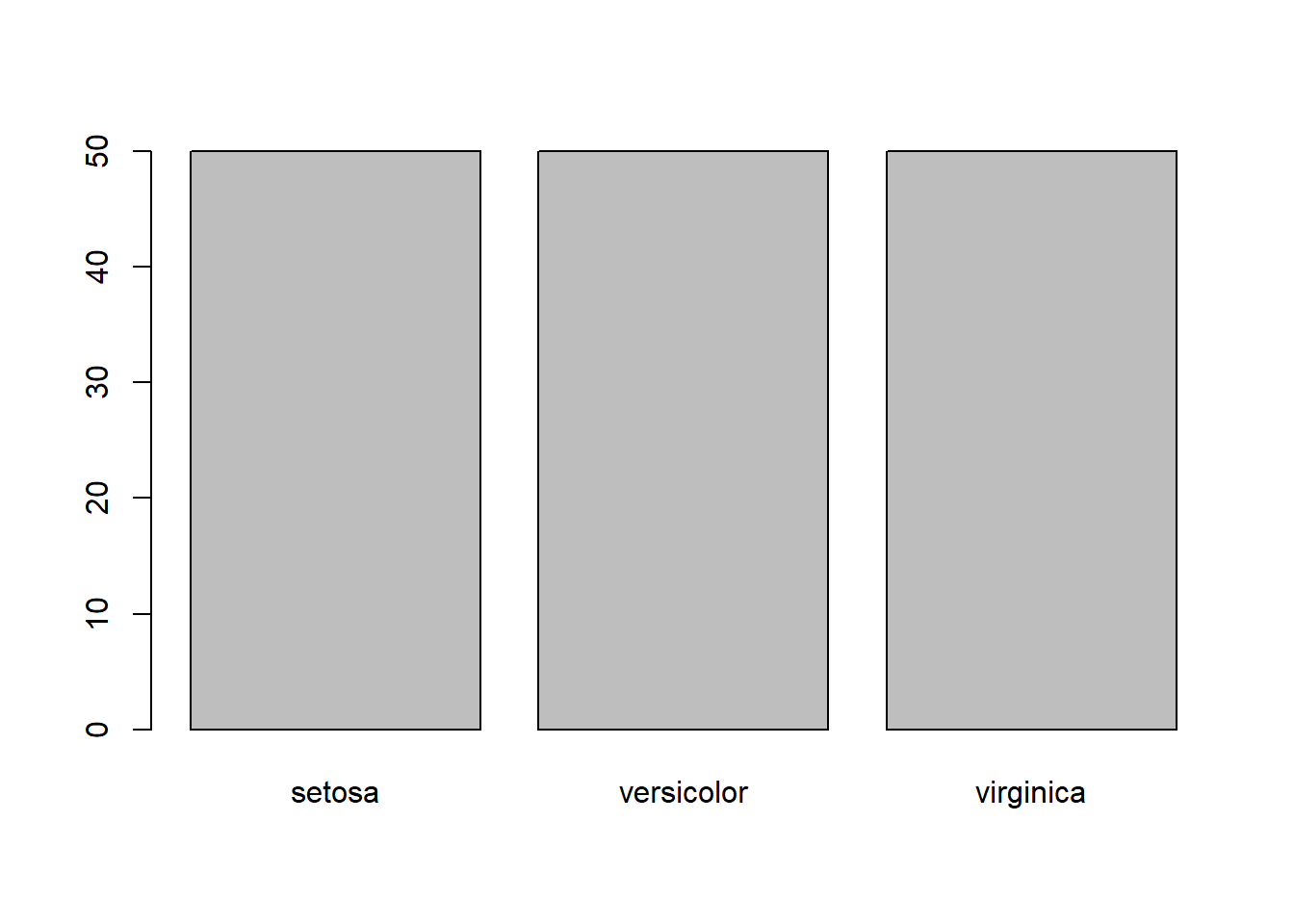There are also parameters to refine the barplots. Please see the following code block for details.

barplot(table(data$Species), #the counts of all classes names = c("setosa", "versicolor", "virginica"), #the names of all bars xlab = "Species", #the name of x axis main = "Barplot of Species", #the title of the barplot ylim = c(0,60), #the range of y axis col = c("darkblue", "darkred", "darkgreen"), #the colors of all bars border = "gold")#the color of the borders of the bars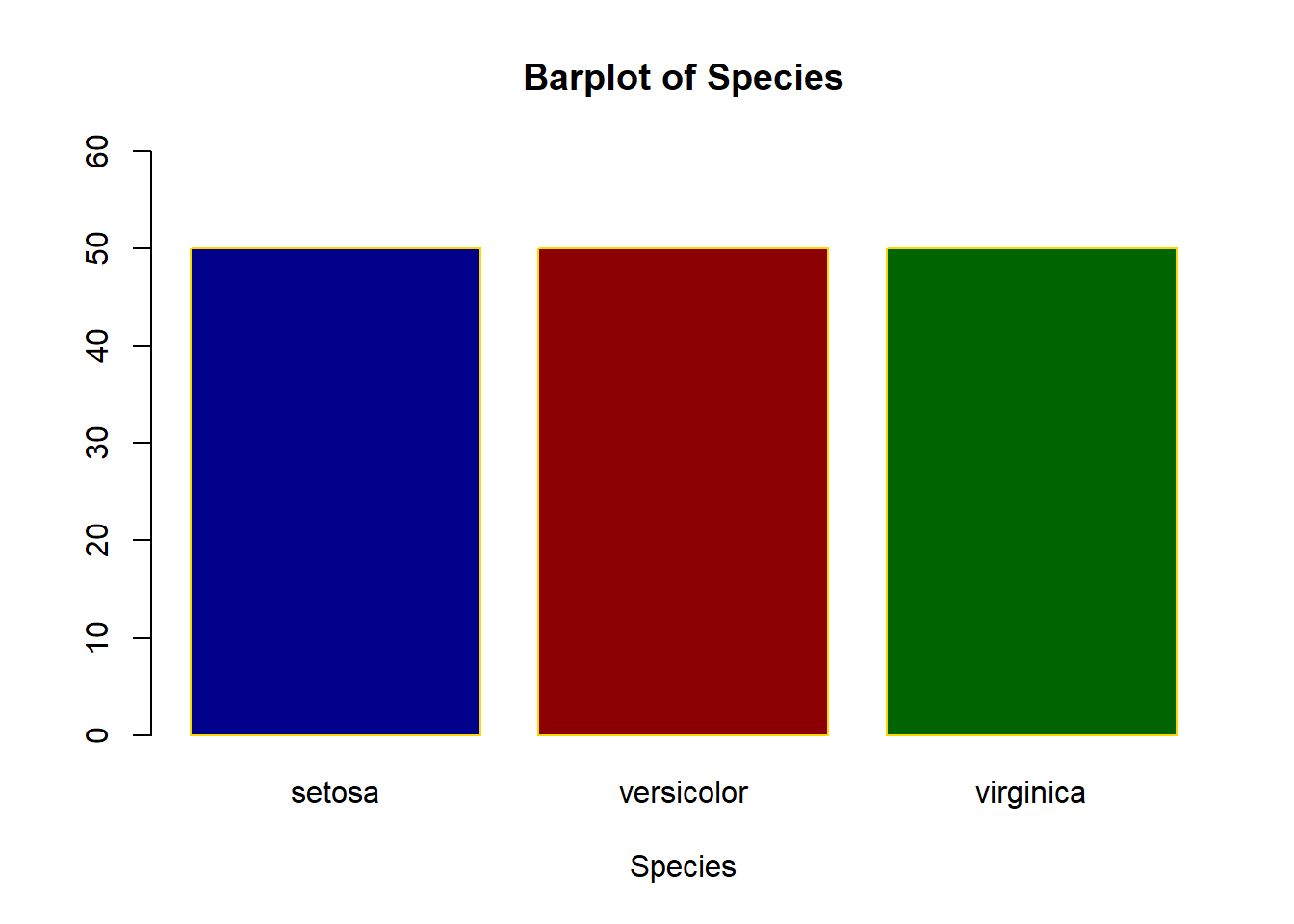If you woule like to rotate the bar plot, you can set the parameter “horiz”. barplot(table(data$Species), #the counts of all classes
names = c("setosa", "versicolor", "virginica"), #the names of all bars
ylab = "Species", #the name of y axis
main = "Barplot of Species", #the title of the barplot
xlim = c(0,60), #the range of x axis
horiz = T,
col = c("darkblue", "darkred", "darkgreen"), #the colors of all bars
border = "gold")#the color of the borders of the bars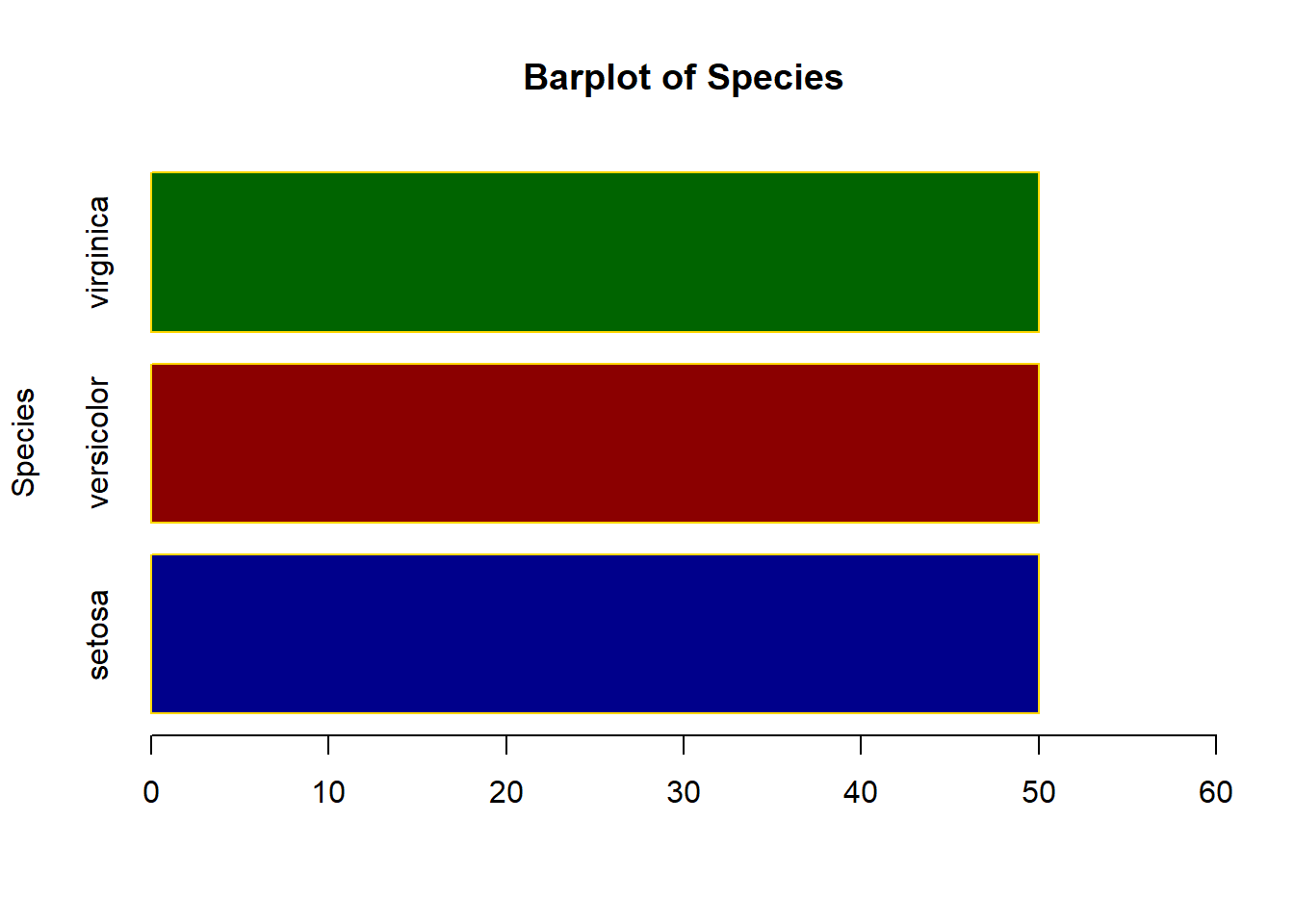#### Boxplots

We can use boxplots to display the relationship of a categorical variable and a numeric variable.

Boxplot can be used to show the distribution of a numeric data set in some sense.

boxplot(data$Sepal.Length)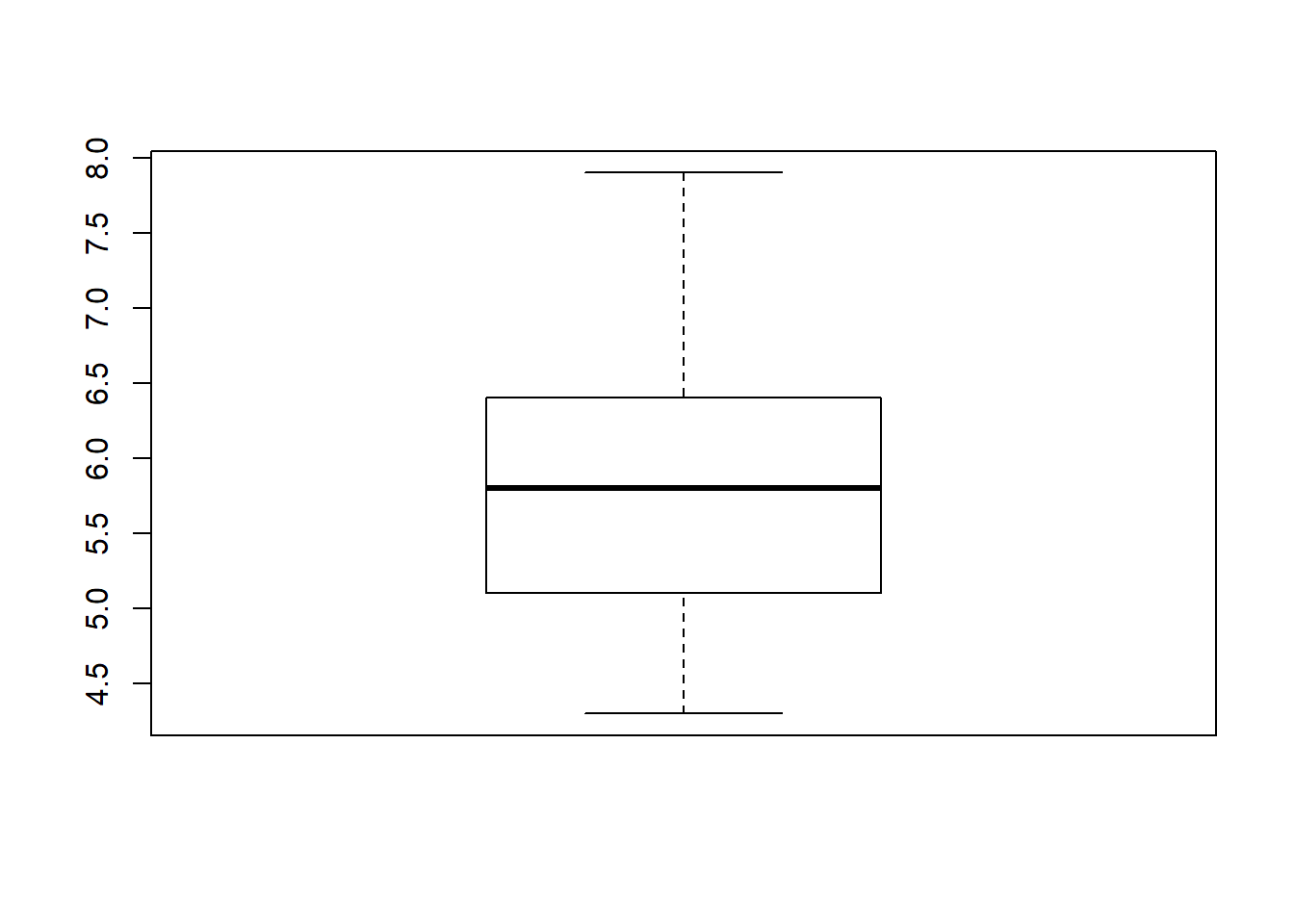The boxplot can also reflect the data of different classes simultaneously. boxplot(Sepal.Length ~ Species, data = data)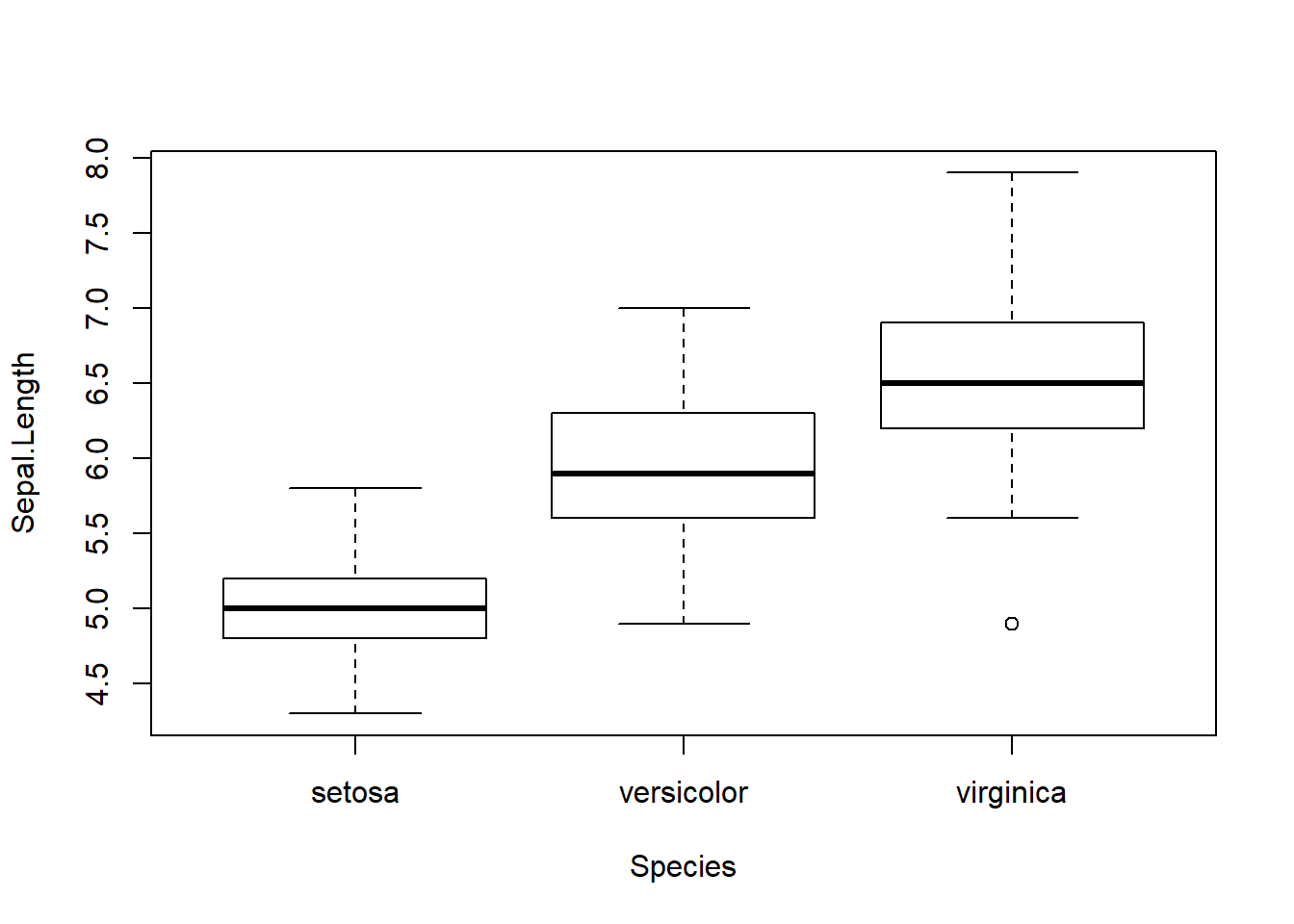The x axis is classified by the species, and boxplot of the Sepal.Length of each sepicies are laid in order. Inputting more parameters can refine the boxplot. The following is an example: boxplot(Sepal.Length ~ Species, data = data, xlab = "Species", #the name of x axis ylab = "Sepal Length", #the name of y axis main = "Sepal Lengh vs Species", #the title of the boxplot col = "grey90", #the color of the fillings in the box border = "darkblue") #the color of the border of the boxplot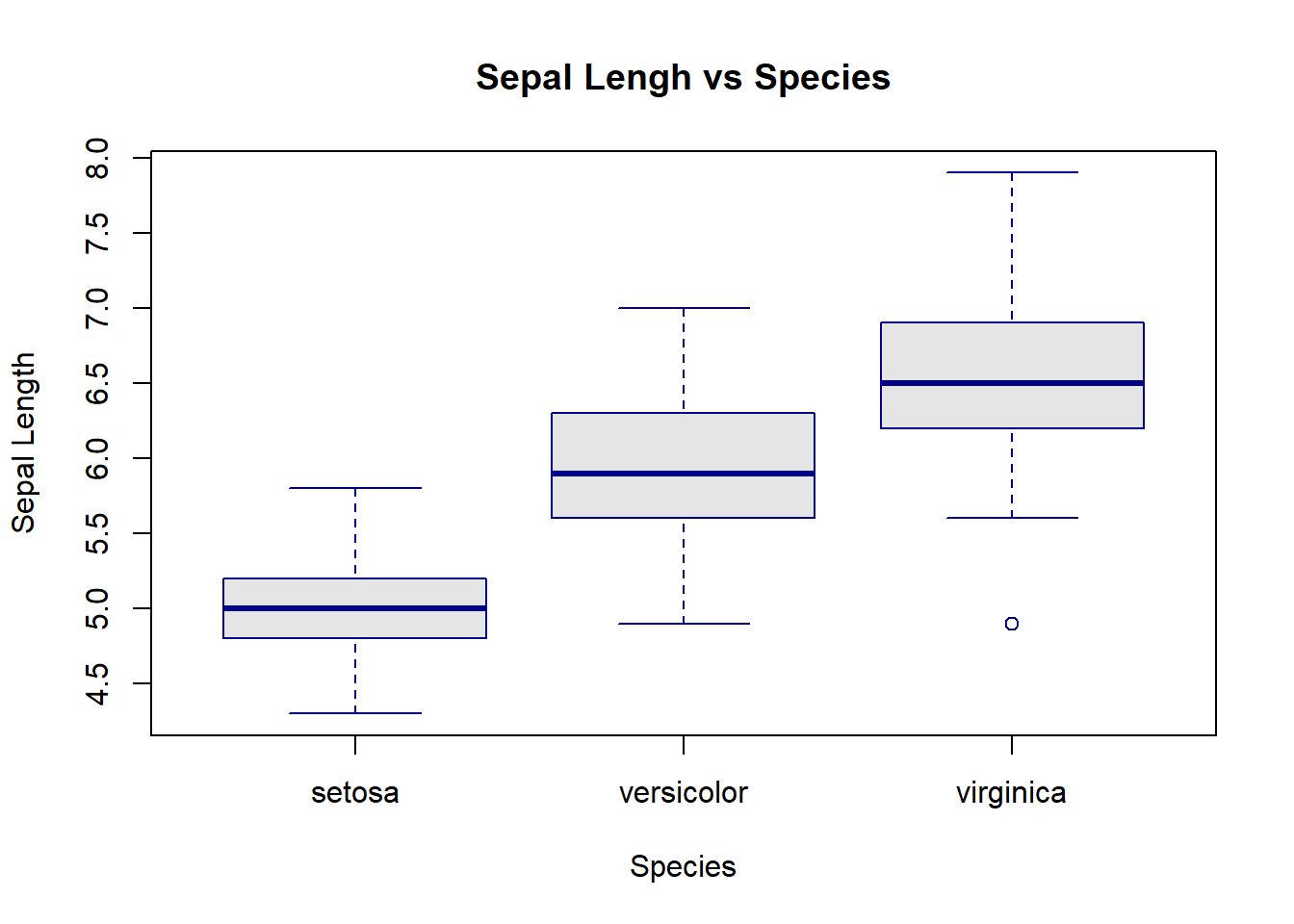#### Scatterplots If only the data of one variable is input, the x axis will be the index of each data point automatically. plot(data$Sepal.Length)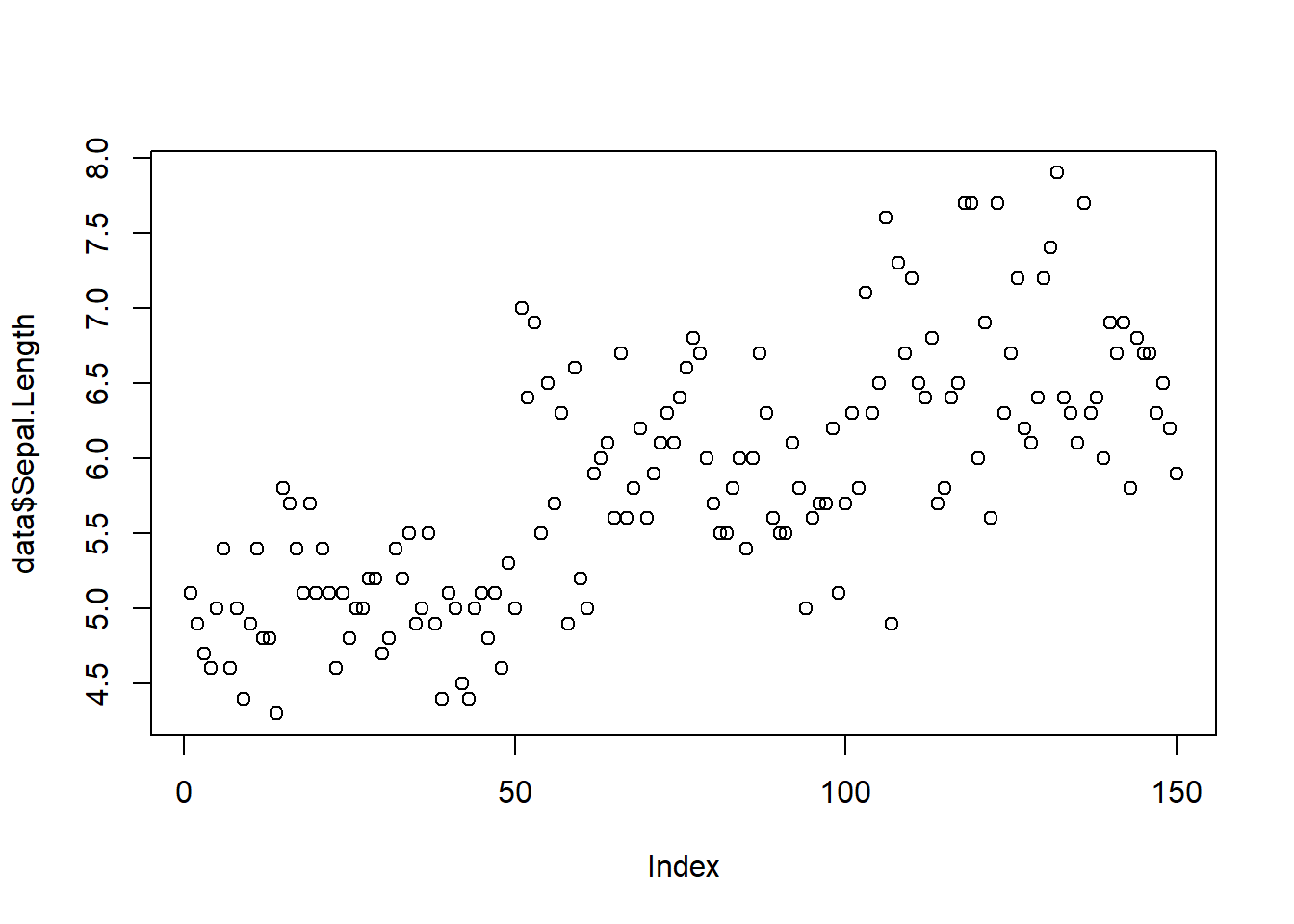Scatterplot can also reflect the relationship of two variables. In the following example, the x and y coordinates of each point represent its sepal width and sepal length respectively.

plot(Sepal.Length ~ Sepal.Width, data = data)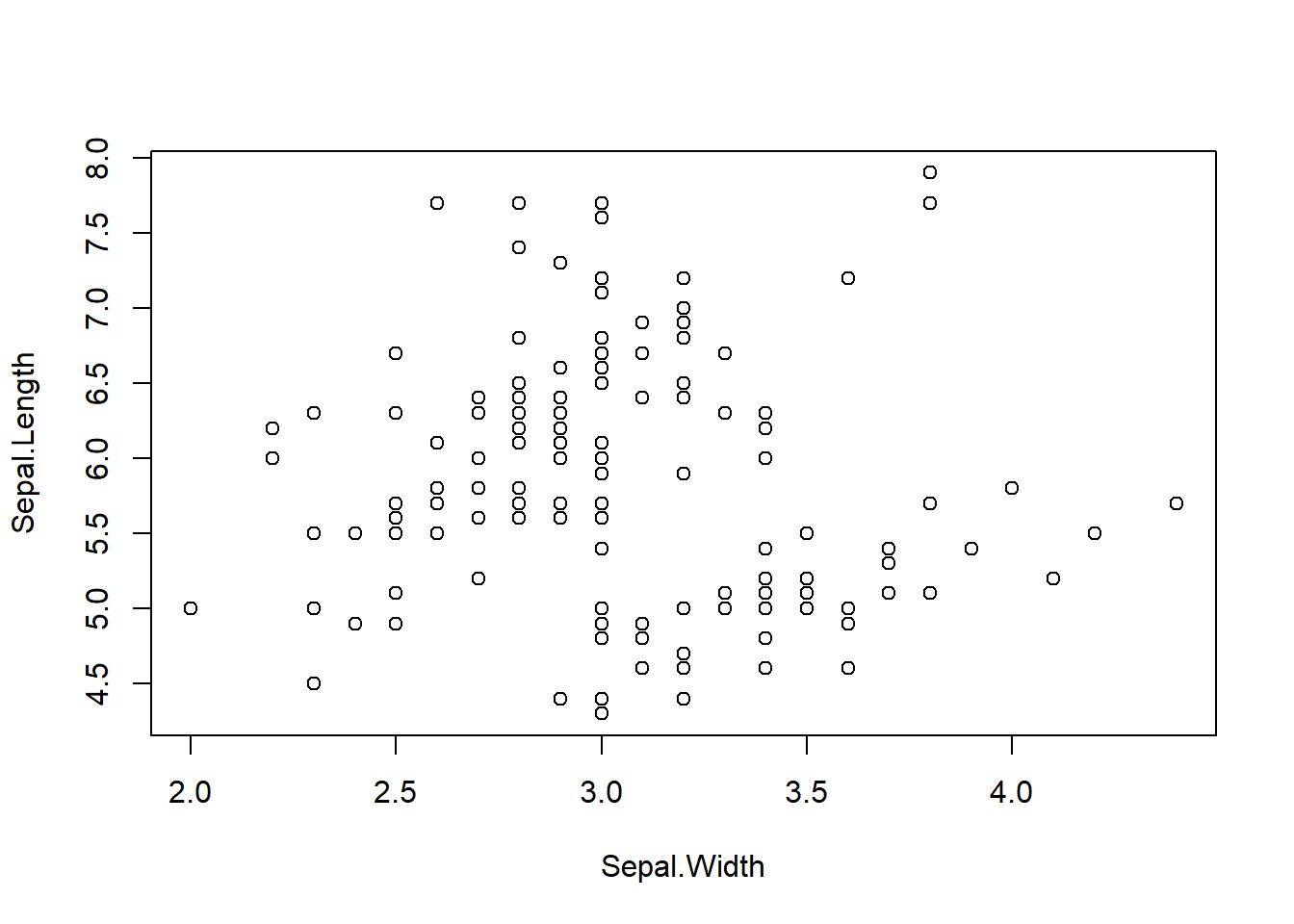plot(data$Sepal.Width, data$Sepal.Length)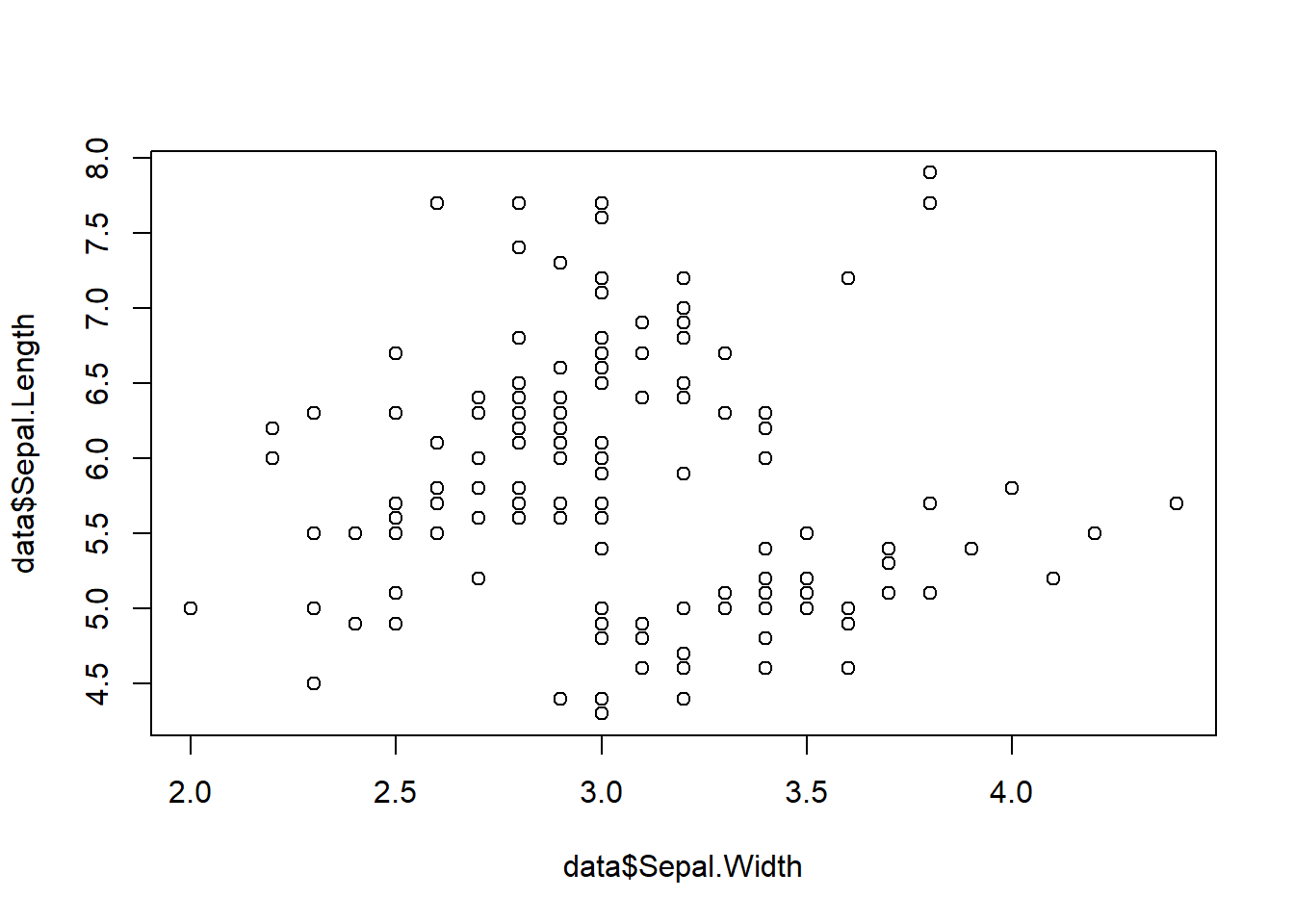#The two ways above are equivalent.
plot(Sepal.Length ~ Sepal.Width, data = data,
xlab   = "Sepal Width", #the name of x axis
ylab   = "Sepal Length", #the name of y axis
main   = "Sepal Lengh vs Sepal Width", #the title of the plot
col    = "darkblue") #the color of the points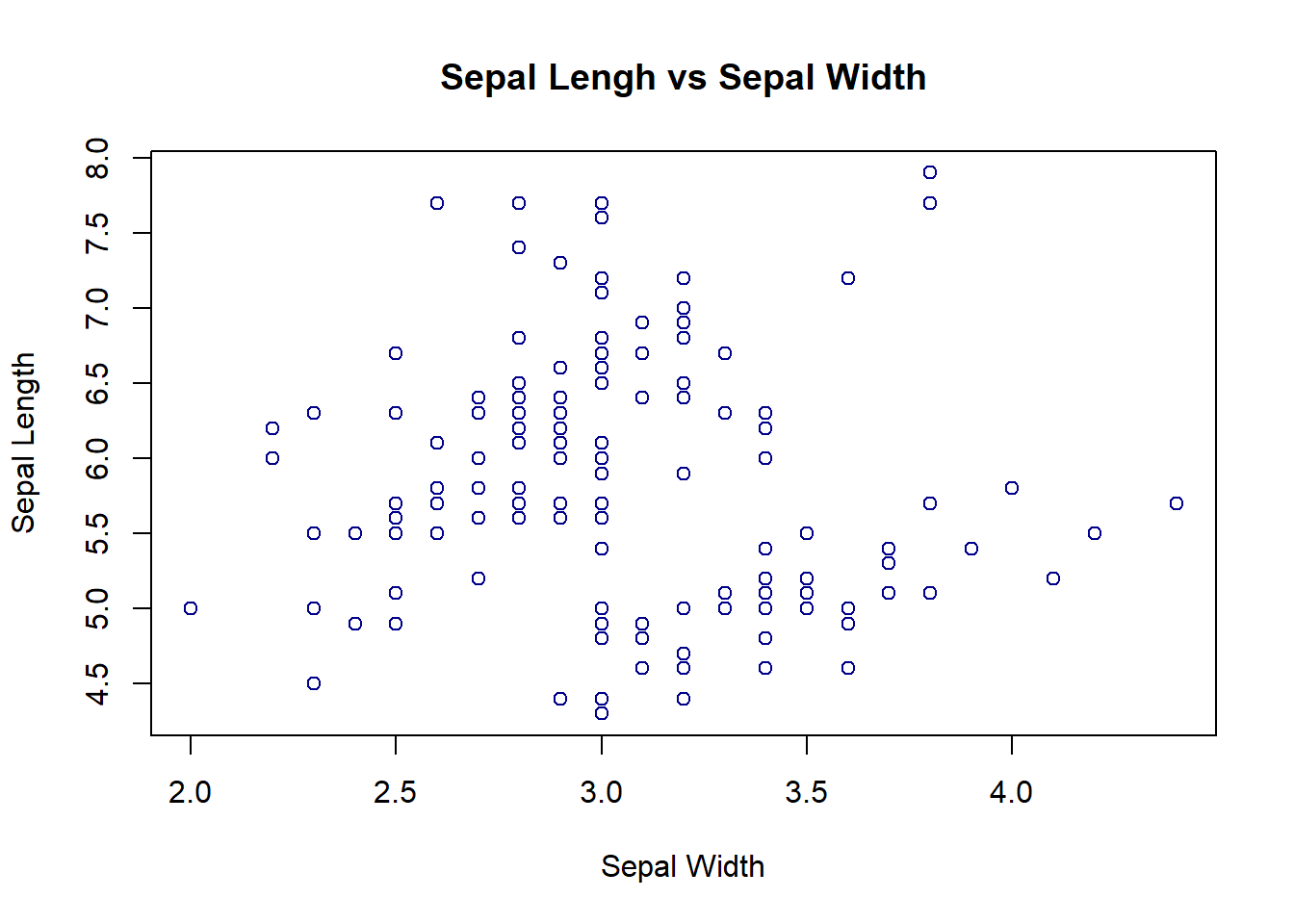#### Line plots

In the “plot” function, setting the parameter “type” as “l” helps return a line plot.

plot(data\$Sepal.Length, #the data
type = "l", #the type of the plot is line plot
col = "darkblue", #the color of the line
lwd = 1, #the width of the line
lty = 1, #the type of the line
ylab = "Sepal Length", #the name of y axis
main = "Sepal Length") #the title of the line plot